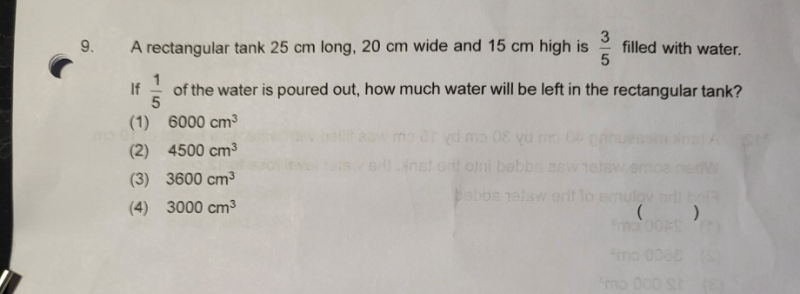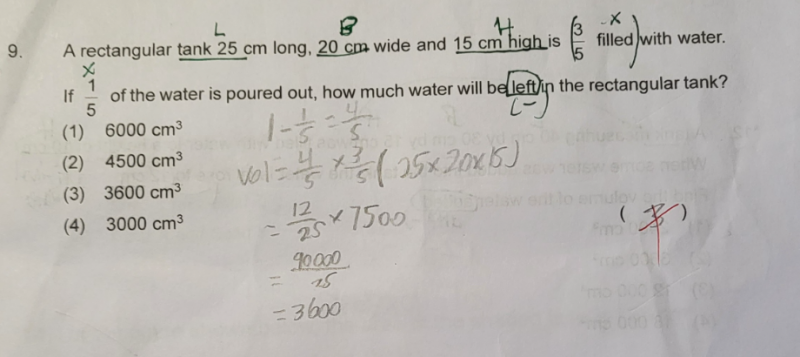# QuestionI went thru the question again, and think the answer is correct. Not sure why it is marked wrong.

3/5 of the tank is filled with water. 1/5 of the water  was poured away. So we can’t remove the 3/5 in calculation of the remaining water.

Unless 1/5 of the water was poured away is interpreted as 1/5 of the volume of the tank  was poured away, in this case,

the amount of water poured away is 1/5 * 25 * 20 * 15 = 1500 cm³

Orginal volume of water in the tank is 3/4 * 25 * 20 * 15 = 4500 cm³

Then the water remained = 4500 – 1500 = 3000 cm³

This doesn’t make sense to me as 1/5 of the water was poured away must refer to  1/5 of the volume of original amount of water in the tank and not 1/5 of the full volume of the tank.

0 Replies 2 Likes ✔Accepted Answer

If 1/5 of the water is poured out, 1 – 1/5 = 4/5 of the water will be left.

So volume of the water left = 4/5 * 3/5 * 25 * 20 * 15 = 3600 cm³I went thru the question again, and think the answer is correct. Not sure why it is marked wrong.

3/5 of the tank is filled with water. 1/5 of the water  was poured away. So we can’t remove the 3/5 in calculation of the remaining water.

Unless 1/5 of the water was poured away is interpreted as 1/5 of the volume of the tank  was poured away, in this case,

the amount of water poured away is 1/5 * 25 * 20 * 15 = 1500 cm³

Orginal volume of water in the tank is 3/4 * 25 * 20 * 15 = 4500 cm³

Then the water remained = 4500 – 1500 = 3000 cm³

This doesn’t make sense to me as 1/5 of the water was poured away must refer to  1/5 of the volume of original amount of water in the tank and not 1/5 of the full volume of the tank.

0 Replies 2 Likes ✔Accepted Answer